# How to use OR function in Microsoft Excel

In this article, we will learn about OR function in Microsoft Excel.

It is one of Excel’s logical functions and it returns “TRUE” if any one condition is TRUE and FALSE. If all the conditions are false OR function only returns Boolean values in the cell. The function returns #VALUE error if no logical values are found.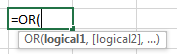OR function takes up to 255 logical arguments, where the 1st argument is mandatory to be entered while rest are optional.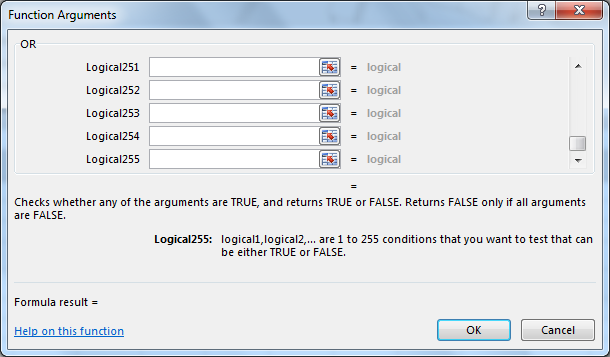Let’s take examples and understand:-

We have taken different examples to understand this function in detail.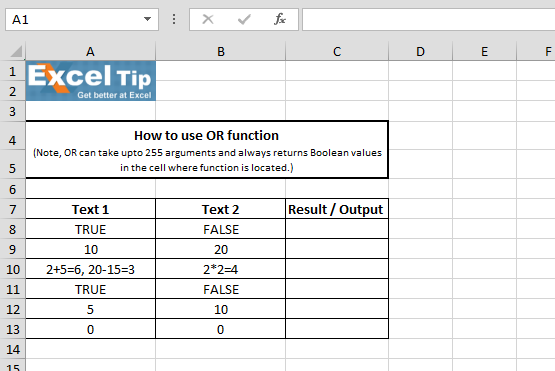1st Example:-

In this example, we will check for two cell’s value.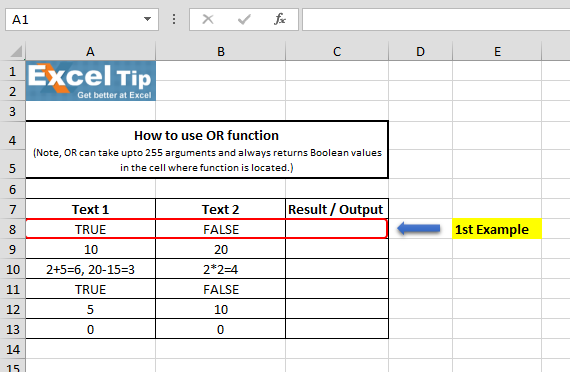Follow the steps given below:-

• Enter the function in cell C8
• =OR(A8,B8), Press Enter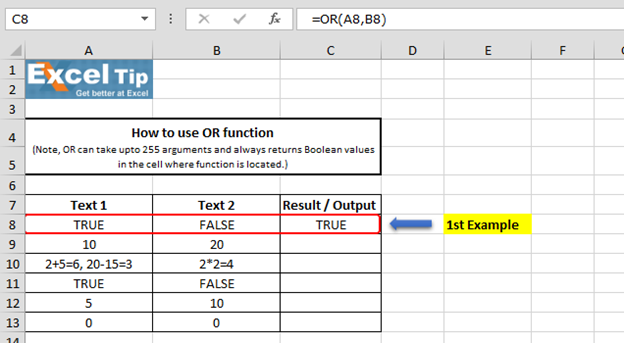• The function has returned TRUE, because 1st argument is TRUE

2nd Example:-

In this example, we will ensure that 1st argument should be greater than 5 and second argument should be less than 25. What will happen when criteria matches and when they do not match?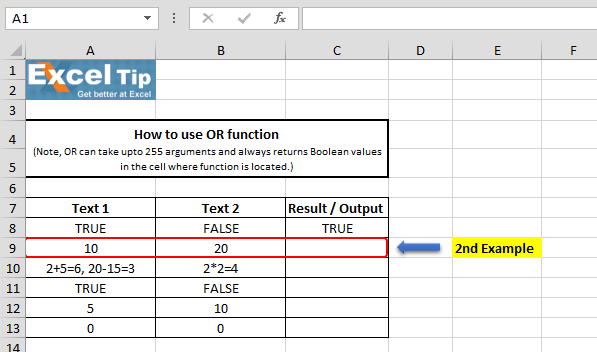Follow the steps given below:-

• Enter the function in cell C9
• =OR(A9>5,B9<25), Press Enter

Result when both criteria are matching:-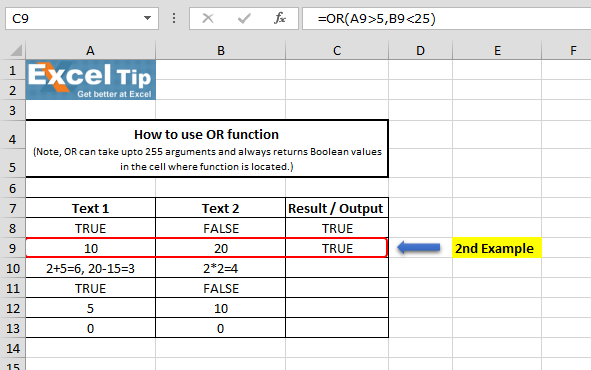Result when one of the criterion matches: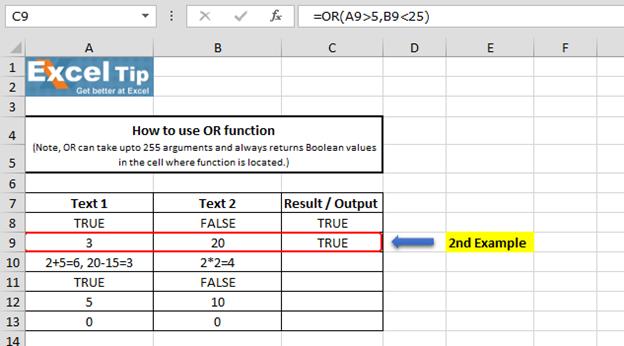Result when both the criteria does not match: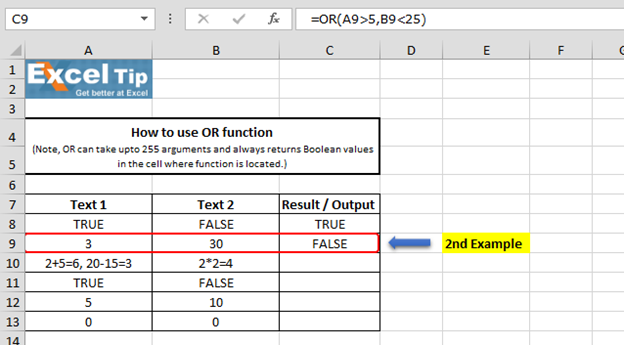It clearly means when any one criterion will match, then function returns TRUE.

3rd Example:-

In this example, we’ll tell excel to do three logical tests and return if any one condition is true.Follow the steps given below:-

• Enter the function in cell C10
• =OR(2+5=6,20-15=3,2*2=4)
• Press Enter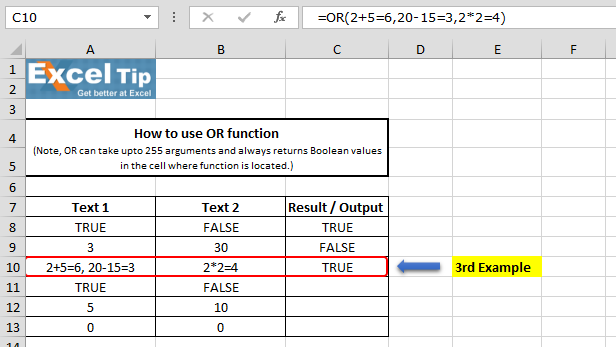Formula Explanation: We have entered 2+5=6 in first argument, 20-15=3 in second and 2*2=4 in third argument and we hit enter. It has returned TRUE because the third condition is matching even if the first two are not.

4th Example:-

In this example, we will learn what happens when we select continuous range.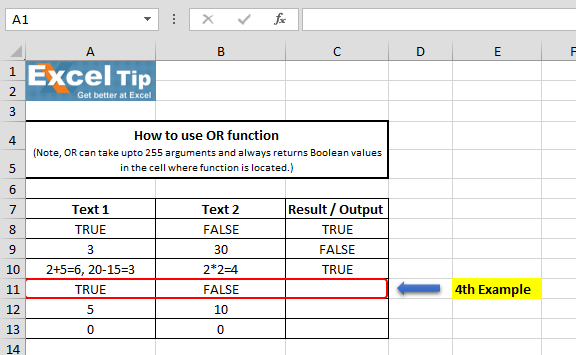Follow the steps given below:-

• Enter the function in cell C11
• =OR(A11:B11), Press Enter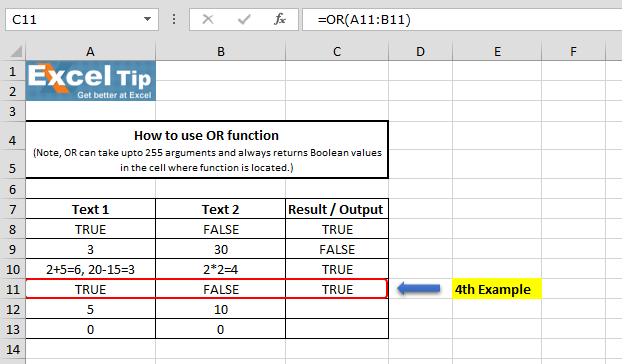The function has returned TRUE, it means we can select any range to check the condition.

5th Example:-

In this example, we will see what happens when we use OR function for numeric value.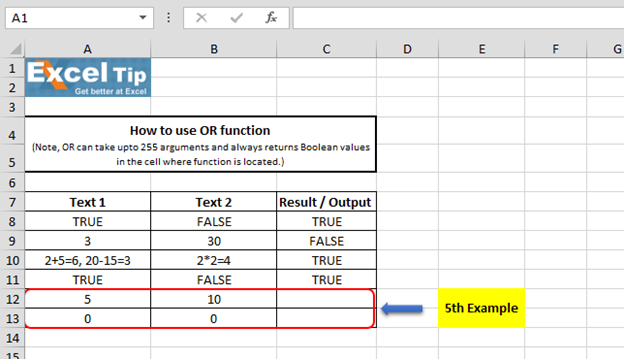Follow the steps given below:-

• Enter the function in cell C12
• =OR(A12,B12), Press Enter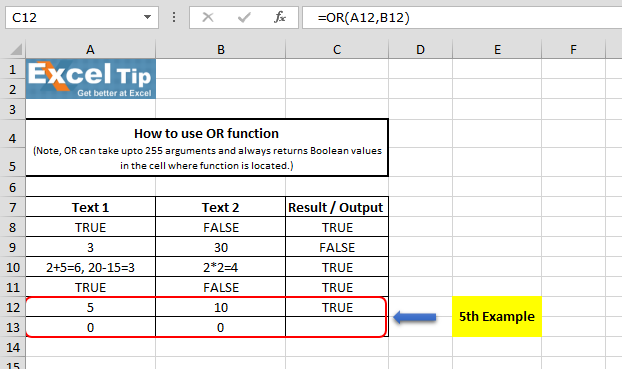The function still returns TRUE whereas both the cells contain different values. It is because OR analyzes the logical arguments, any positive number is by default considered as TRUE.

But what happens when we take 0 in the logical test, the function would return FALSE because “0” is always considered FALSE by OR.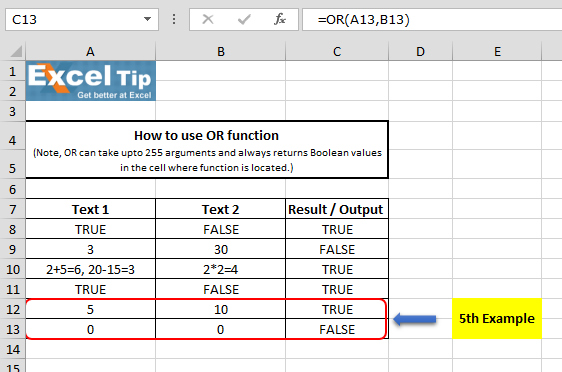So, this is how OR function works in Microsoft Excel.# Video: How to use OR function in Microsoft Excel

Watch the steps in this short video, and the written instructions are above the video

If you liked our blogs, share it with your friends on Facebook. And also you can follow us on Twitter and Facebook.
We would love to hear from you, do let us know how we can improve, complement or innovate our work and make it better for you. Write us at info@exceltip.com

#### Categories

Terms and Conditions of use

The applications/code on this site are distributed as is and without warranties or liability. In no event shall the owner of the copyrights, or the authors of the applications/code be liable for any loss of profit, any problems or any damage resulting from the use or evaluation of the applications/code.